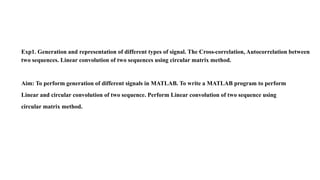Anúncio

# EE306_EXP1.pptx

9 de Nov de 20221 de 18
Anúncio

### EE306_EXP1.pptx

1. Exp1. Generation and representation of different types of signal. The Cross-correlation, Autocorrelation between two sequences. Linear convolution of two sequences using circular matrix method. Aim: To perform generation of different signals in MATLAB. To write a MATLAB program to perform Linear and circular convolution of two sequence. Perform Linear convolution of two sequence using circular matrix method.
Anúncio